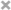### 什么情况下需要布隆过滤器？

• 字处理软件中，需要检查一个英语单词是否拼写正确
• 在 FBI，一个嫌疑人的名字是否已经在嫌疑名单上
• 在网络爬虫里，一个网址是否被访问过
• yahoo, gmail等邮箱垃圾邮件过滤功能

• 数组
• 链表
• 树、平衡二叉树、Trie
• Map (红黑树)
• 哈希表

### 哈希函数### 布隆过滤器介绍

• 巴顿.布隆于一九七零年提出
• 一个很长的二进制向量 （位数组）
• 一系列随机函数 (哈希)
• 空间效率和查询效率高
• 有一定的误判率（哈希表是精确匹配）

### 布隆过滤器原理### 布隆过滤器添加元素

• 将要添加的元素给k个哈希函数
• 得到对应于位数组上的k个位置
• 将这k个位置设为1

### 布隆过滤器查询元素

• 将要查询的元素给k个哈希函数
• 得到对应于位数组上的k个位置
• 如果k个位置有一个为0，则肯定不在集合中
• 如果k个位置全部为1，则可能在集合中

### 布隆过滤器实现

``````# Implement a simple bloom filter with murmurhash algorithm.
# Bloom filter is used to check wether an element exists in a collection, and it has a good performance in big data situation.
# It may has positive rate depend on hash functions and elements count.

BIT_SIZE = 5000000

class BloomFilter:

def __init__(self):
# Initialize bloom filter, set size and all bits to 0
bit_array = bitarray(BIT_SIZE)
bit_array.setall(0)

self.bit_array = bit_array

# Add a url, and set points in bitarray to 1 (Points count is equal to hash funcs count.)
# Here use 7 hash functions.
point_list = self.get_postions(url)

for b in point_list:
self.bit_array[b] = 1

def contains(self, url):
# Check if a url is in a collection
point_list = self.get_postions(url)

result = True
for b in point_list:
result = result and self.bit_array[b]

return result

def get_postions(self, url):
# Get points positions in bit vector.
point1 = mmh3.hash(url, 41) % BIT_SIZE
point2 = mmh3.hash(url, 42) % BIT_SIZE
point3 = mmh3.hash(url, 43) % BIT_SIZE
point4 = mmh3.hash(url, 44) % BIT_SIZE
point5 = mmh3.hash(url, 45) % BIT_SIZE
point6 = mmh3.hash(url, 46) % BIT_SIZE
point7 = mmh3.hash(url, 47) % BIT_SIZE

return [point1, point2, point3, point4, point5, point6, point7]
```
``````